## The Los Alamos fission yield evaluation pipeline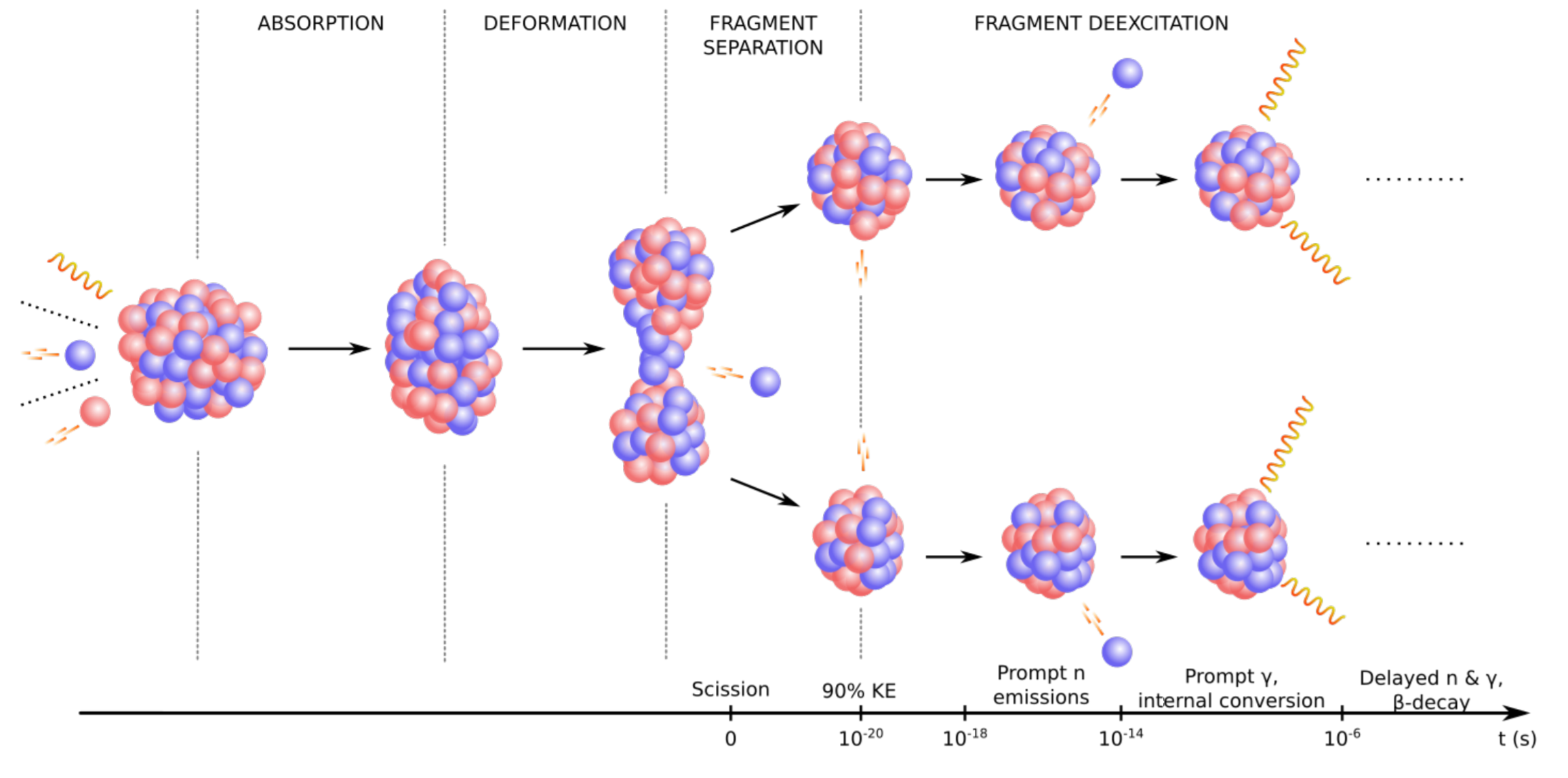LA-UR-19-29974

### Matthew Mumpower

FPY workshop

Thursday Oct. 3$^{rd}$ 2019Nuclear Data Team
Theoretical Division

## Outline

• Motivation
• What is an evaluation?
• Progress on our evaluation pipeline
• Summary## Why do we need fission yields?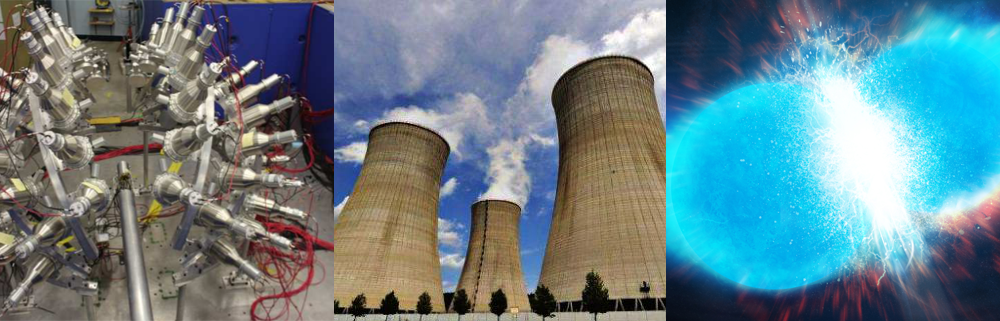Fission yields are needed for a variety of applications

Industrial applications: simulation of reactors, fuel cycles, waste management

Experiments: backgrounds, isotope production with radioactive ion beams (fragmentation)

Science applications: nucleosynthesis, light curve observations

Other Applications: national security, nonproliferation, nuclear forensics

Figure: Chi-Nu detector (left), Reactor cooling towers (middle), Neutron star merger (right)

## What is a fission yield evaluation?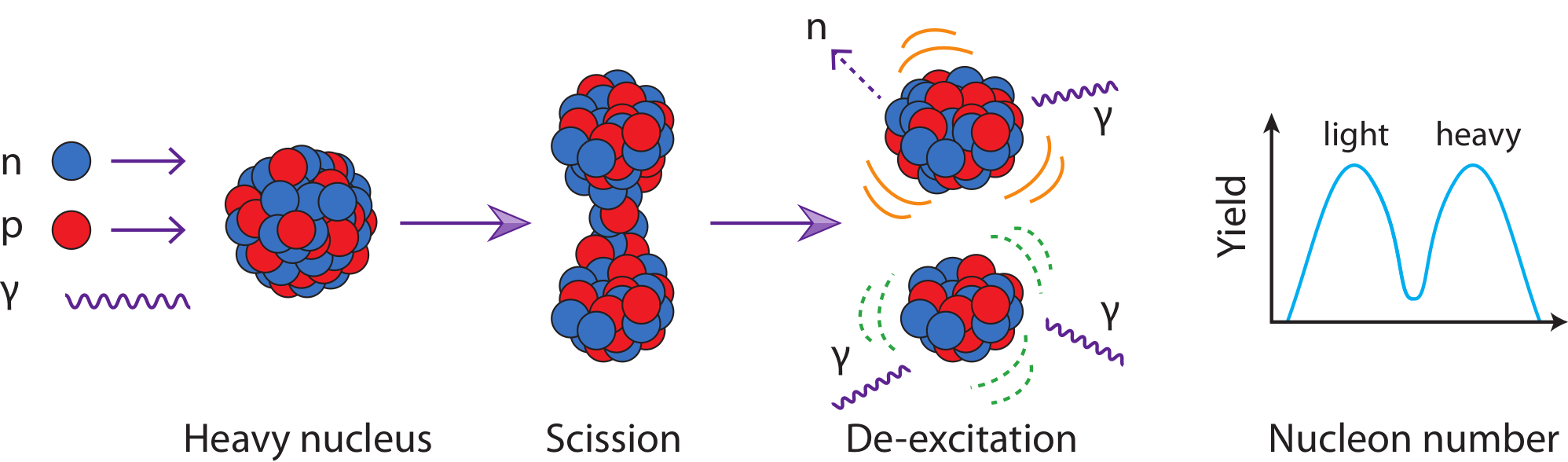An evaluation combines experimental and theoretical knowledge consistent with physical laws

to produce a quantity (in this case fission yields) with verifiable quality.

Should be distributed in an easily accessible form that all case use (e.g. ENDF or GNDS)

for a variety of applications

Theory is limited in accuracy & measured data is limited in scope

Figure by M. Mumpower

## Current status of fission yield evaluations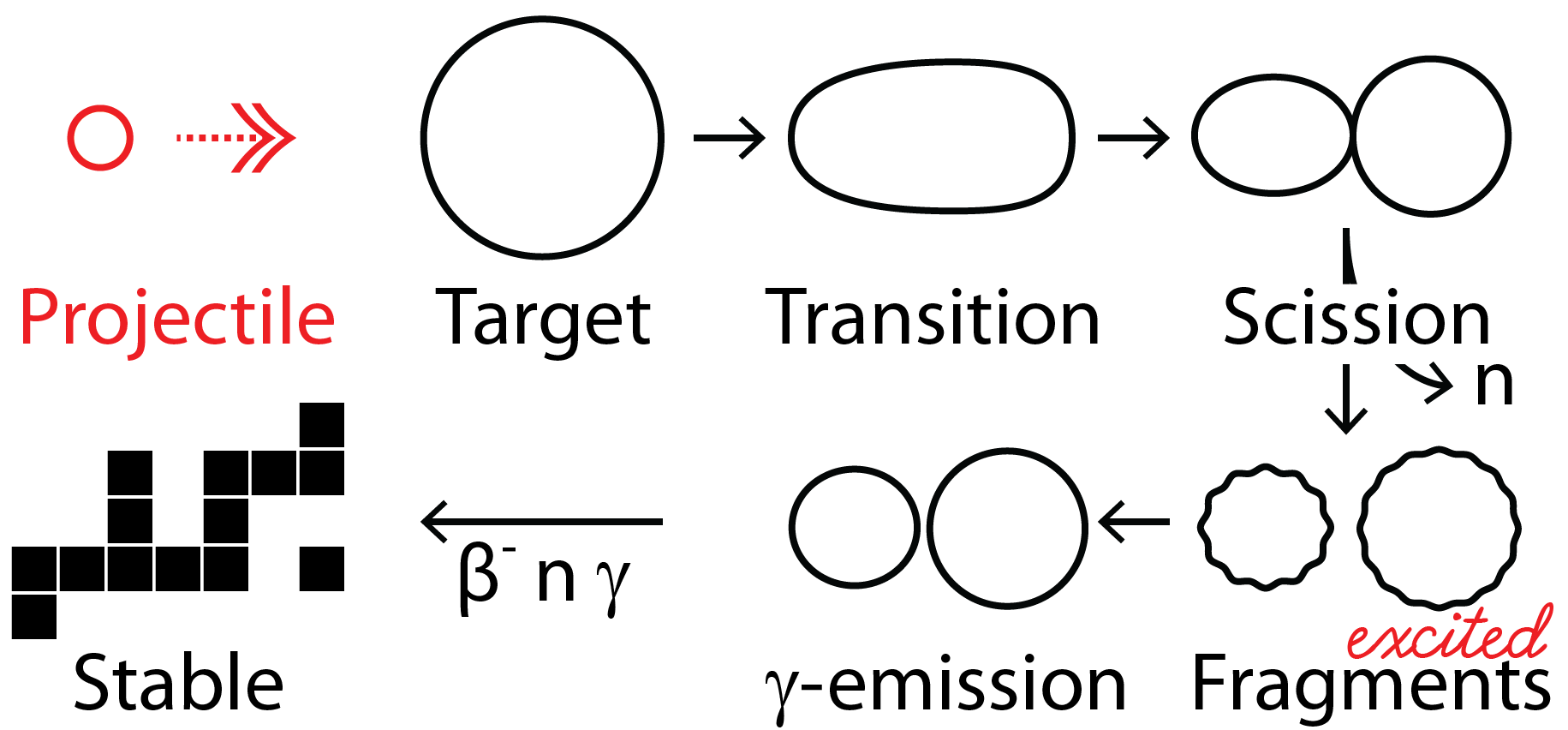The last update to ENDF fission yield evaluation was in the 1990's

Other evaluations exists such as (JEFF, JENDL, etc.)

We want to improve several key areas:

• improve continuous energy dependent information
• provide consistency between yields, n's & $\gamma$'s and decay data
• more complete uncertainties

Figure by M. Mumpower

## The Los Alamos Fission Yield Evaluation

Purpose: to provide a modern evaluation of fission yields

With a focus on consistency between yields, n's & $\gamma$'s, and decay data

This includes:

• independent yield (after prompt particle emission)
• cumulative yield (after all decays)

We also seek to expand continuous energy dependent information

Auxiliary information:

primary yield (before any prompt particle emission)

Particle spectra and multiplicities (n's & $\gamma$'s)

## The LANL Fission Evaluation Pipeline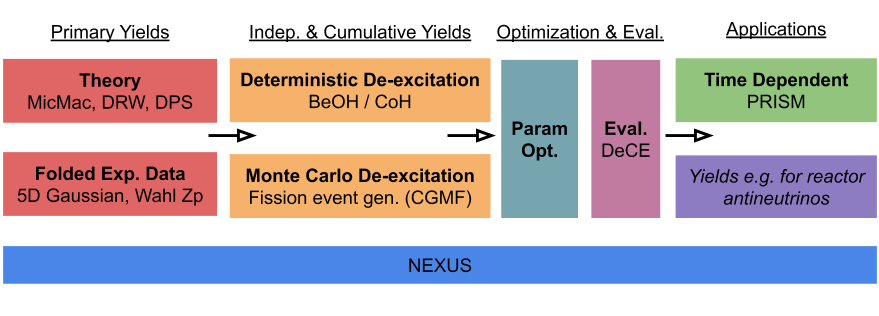Our current workflow is a combination of many distinct codes

woven together by a Python3 framework called NEXUS.

MicMac [C++/Python]: nuclear potential energy surface generation

Theoretical calculation of fissioning system based off Finite-Range Liquid-Drop Model

DRW / DPS [C++/Fortran/Python]: primary yield event generators

Theoretical calculation of fission dynamics based off stochastic random walk

BeOH / CGMF [C++]: de-excitation modeling both deterministically and via Monte Carlo

Follows the statistical decay of excited fragments; IY, CY, isomer ratios

KALMAN [Fortran]: Bayesian parameter optimizer

Linear first-order parameter optimzation scheme

DeCE [C++]: evaluation file interaction

Parsing and generation of evaluation files

PRISM [Fortran/Python]: time-ordered information using nuclear reaction network

Generation of cumulative yield; decay heat

## Theoretical methods for fission

We could try to build our model from the ground up using quantum mechanics - this is hard

Energy-density functional theory (DFT) calculations are built on this approach

Can give key physical insight into system of interest

Drawbacks stem from uncertainty in choice of functionals and high computational costs

### Or...

View the nucleus as a liquid drop composed of macroscopic and microscopic terms - less hard

Macroscopic-microscopic methods are based on this approach

Fast and scalable; good for performing global calculations

Drawbacks: these models are not self-consistent; dependent on fine-tuned parameters

Nix & Swiatecki NP 71 (1965) • Schunck & Robledo RPP 116301 (2016)

## A basic picture of fission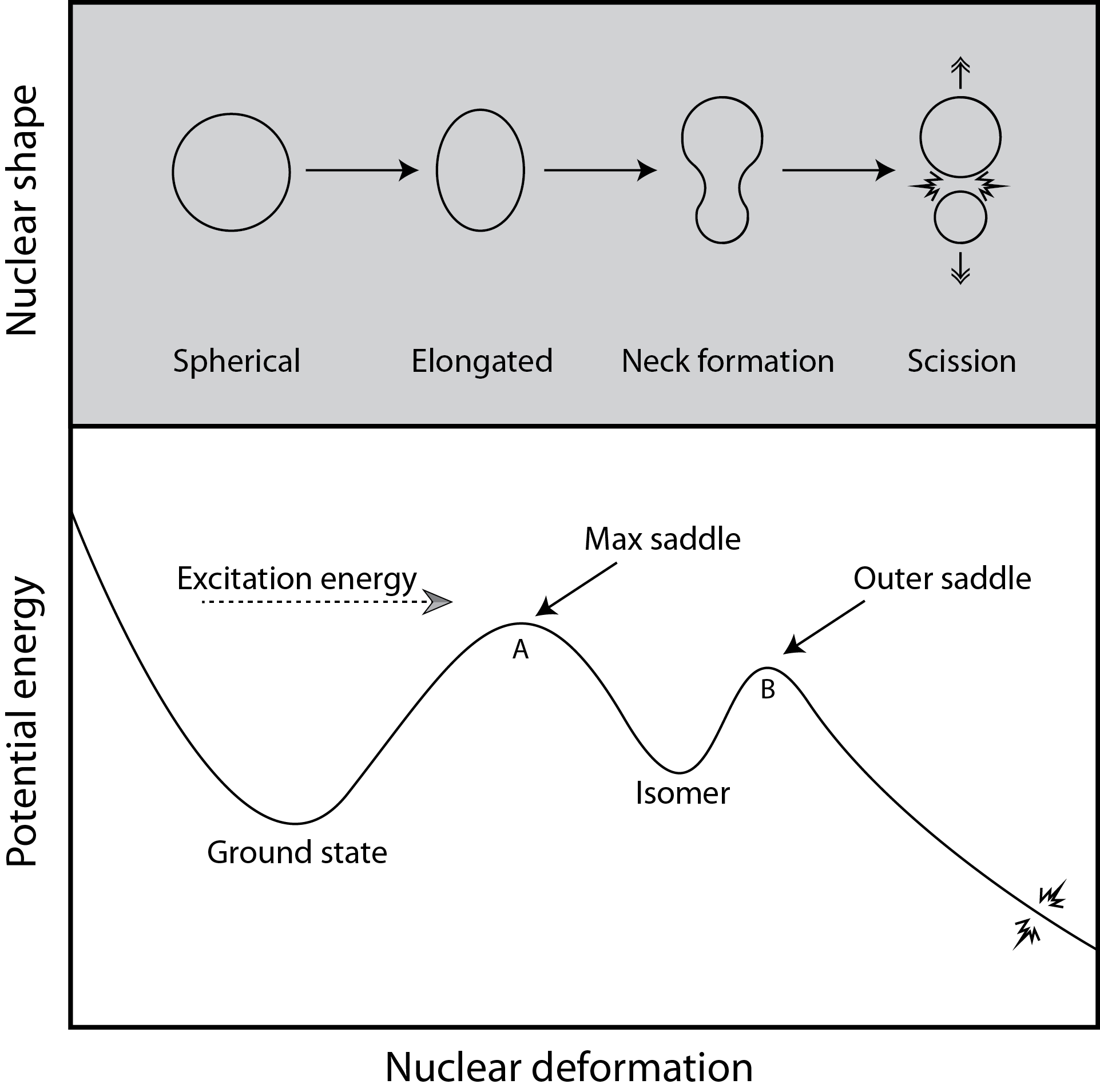Follow progression of the nucleus from compact to highly elongated shapes

Figure from Mumpower et al. in prep. (2019)

## Finite-Range Liquid-Drop Model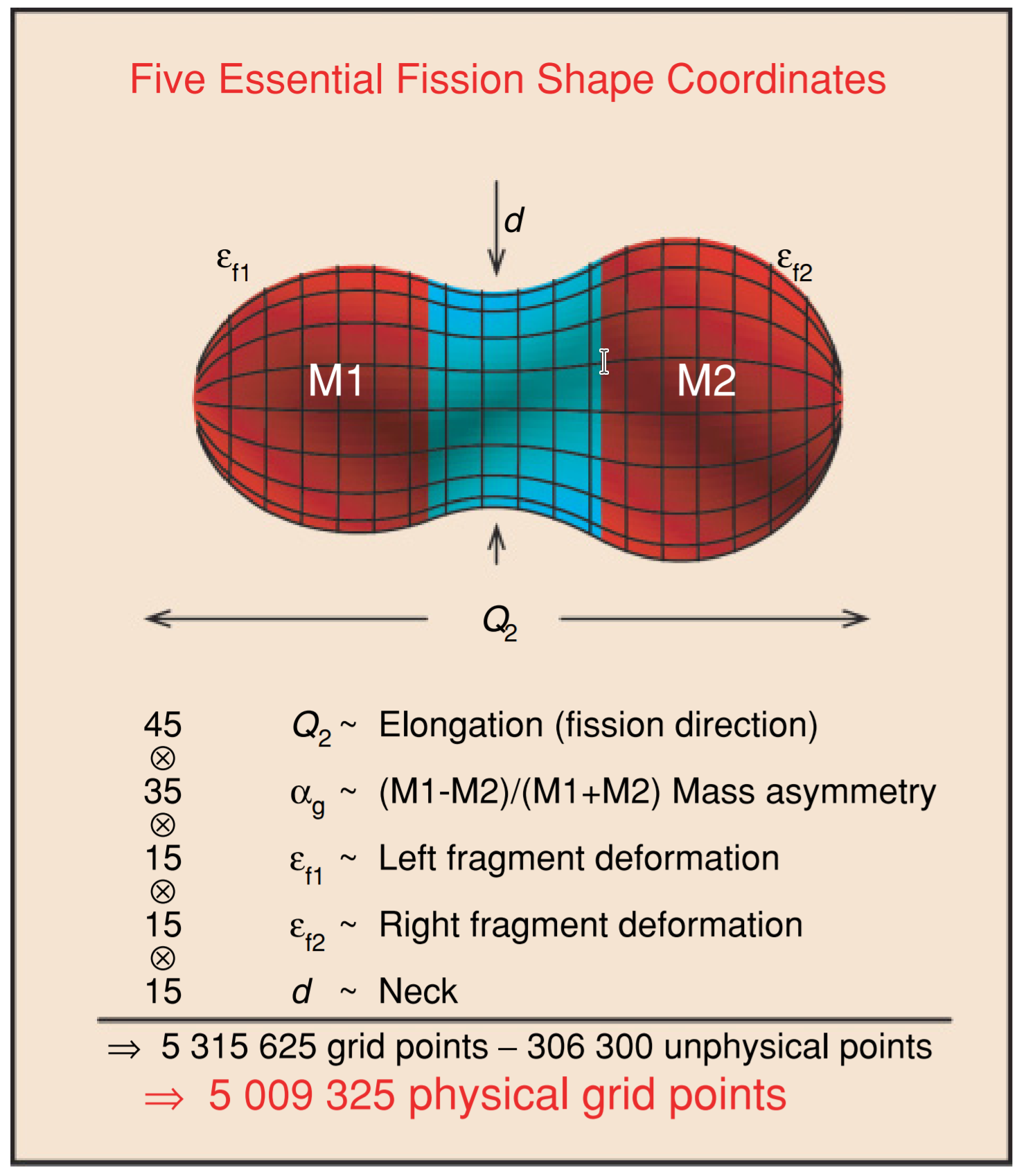Many possible shape degrees of freedom - but we have to isolate the most important

Möller et al. PRC (2009) • Möller et al. PRC (2015)

## Calculation of nuclear potential energy surfaces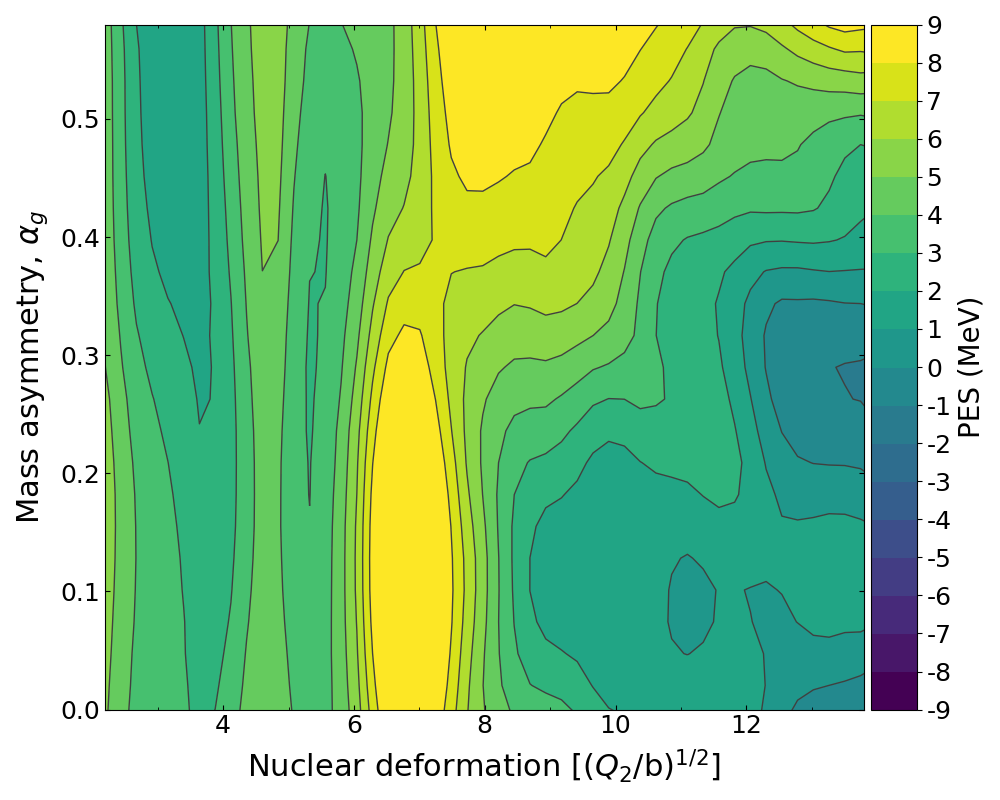Projected potential energy surface from 5 canonical shape parameters of FRLDM

Path to sission is dependent on the trajectory through this complex surface

Nuclear potential energy surface of 236-U

Möller et al. PRC (2015) • Verriere et al. (2019)

## Calculation of fission yields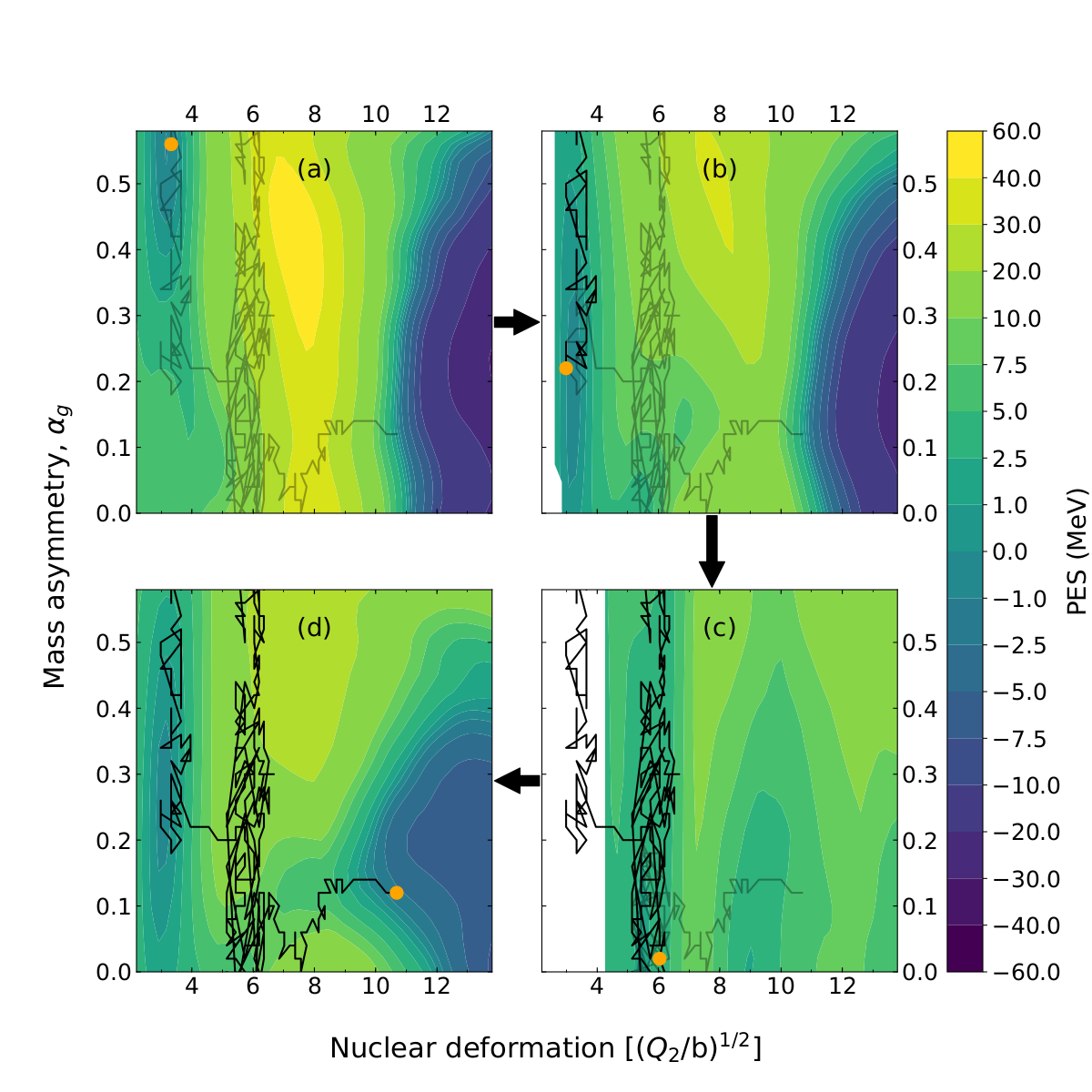Change in nuclear shape acts as a driving force for bulk rearrangement of material

Amounts to random walk across potential energy surface

Randrup et al. PRL (2011) • Randrup et al. PRC (2011) • Randrup et al. PRC (2013) • Mumpower et al. in prep. (2019)

## Theoretical primary yield compared to data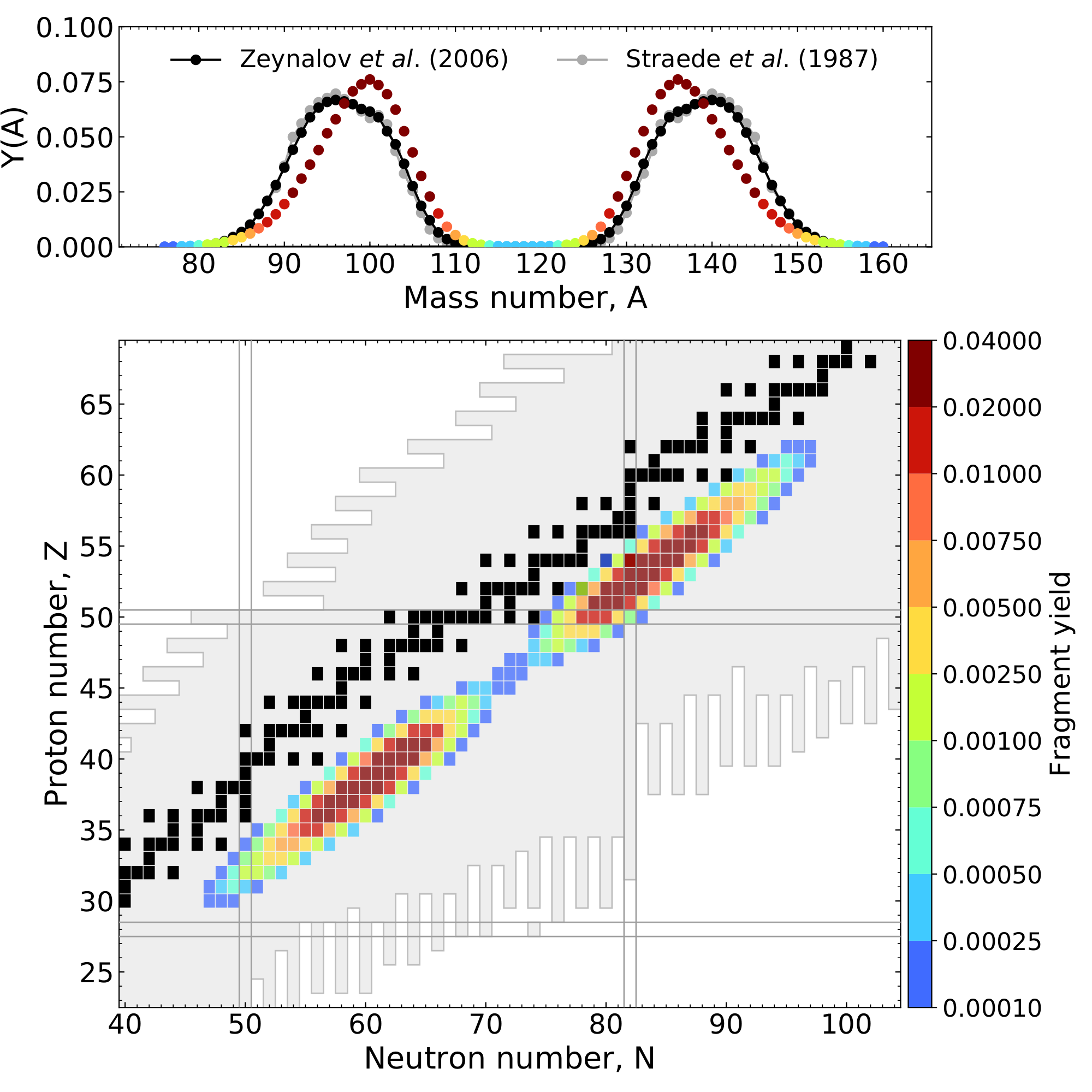Ensemble of fission events leads to the cumulation of the yield curve ($^{235}$U + n$_{\rm{therm}}$)

Relies on geometric splitting argument for the scission configuration

Mumpower et al. in prep. (2019)

## Charge yield with odd-even staggering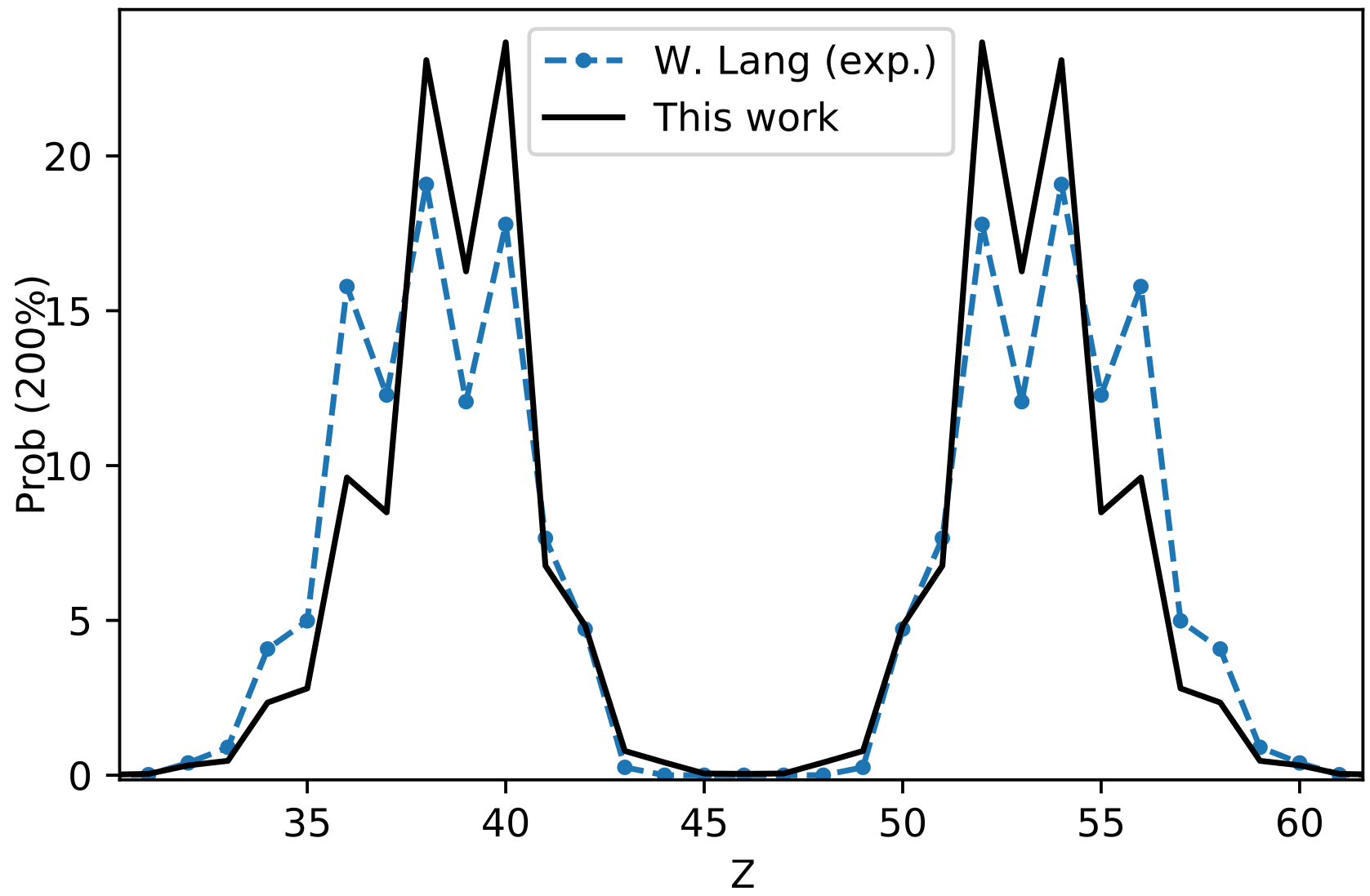We have further enhanced these calculations; moving beyond the geometric picture

First theoretical prediction of odd-even staggering using a particle number projection technique for $^{235}$U + n$_{\rm{therm}}$

Even more improvement when larger quantum basis is used (recall Marc Verriere's talk)

Verriere et al. PRC 100 024612 (2019)

## BeOH: statistical de-excitation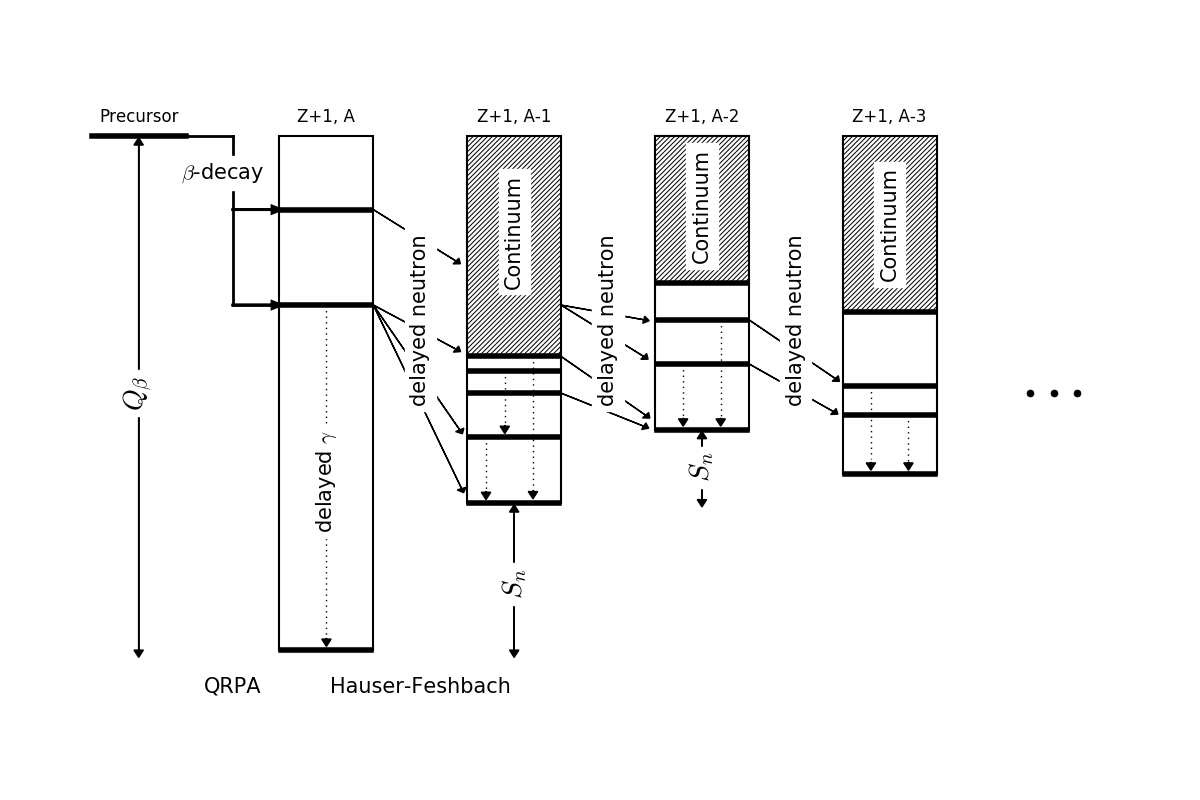Assume Bohr indepdence hypothesis of compound nucleus formation

Can study n, $\gamma$ and fission competition with $\beta$-decay

Used to study $\beta$-delayed neutron emission and multi-chance $\beta$-delayed fission (new decay mode)

Recently has been applied to statistical de-excitation of nascent fission fragments

Kawano et al. PRC (2008) • Mumpower et al. PRC 94 (2016) • Spyrou et al. PRL (2016) • Mumpower et al. ApJ (2018)
Jaffke et al. PRC 97 (2018) • Okumura et al. JNST (2018) • Yokoyama et al. PRC (2019)

## Calculation of independent yield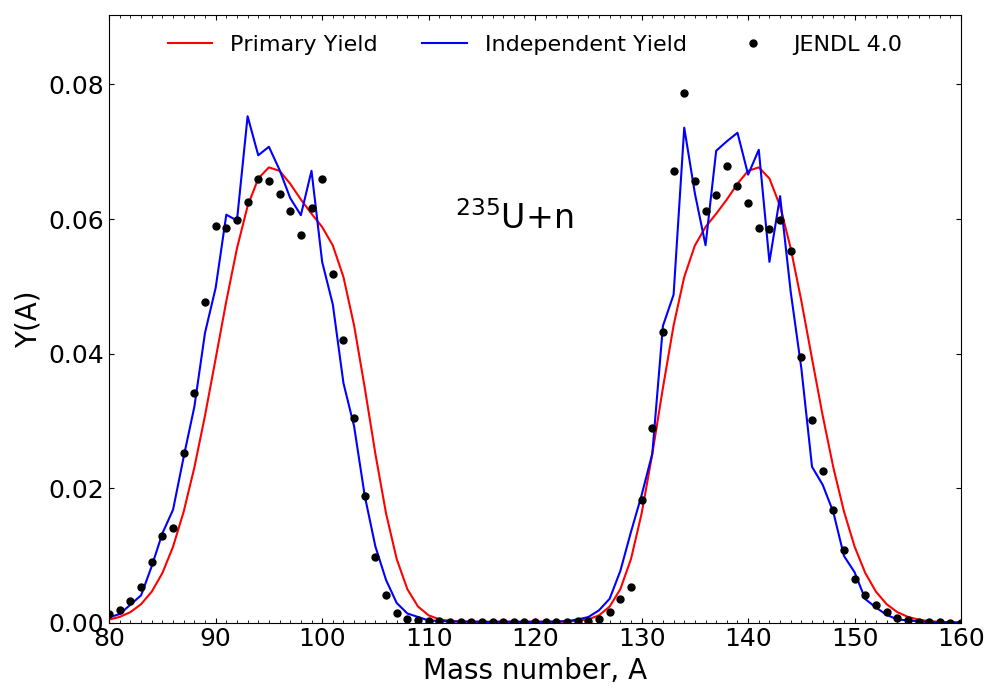Fit data: Hambsch for $^{235}$U + n thermal fission

Primary yield (PY): using 5D Gaussian fitting procedure; charge systematics from Wahl

Independent yield (IY): after prompt neutron and $\gamma$-ray emission using BeOH / HF$^3$D

Wahl LA-13928 (2002) • Jaffke et al. PRC 97 (2018) • Okumura et al. JNST 55 (2018)

## Average prompt neutrons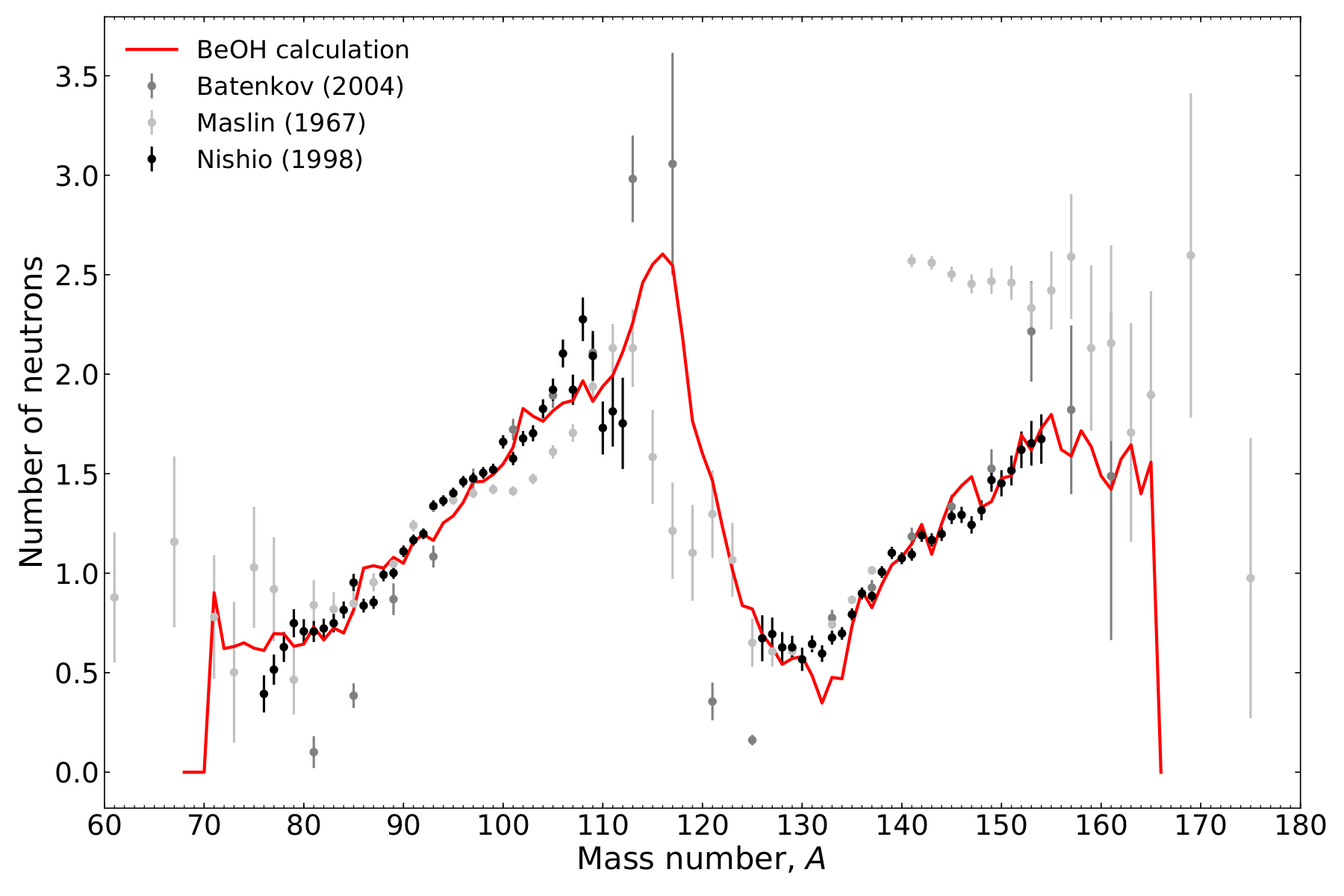Average prompt neutrons emitted as a function of fragment mass number

Critical inputs: yield; total kinetic energy as a function of mass number and excitation energy sharing

Increasing confidence of using these models for our evaluation efforts

## Auxiliary outputs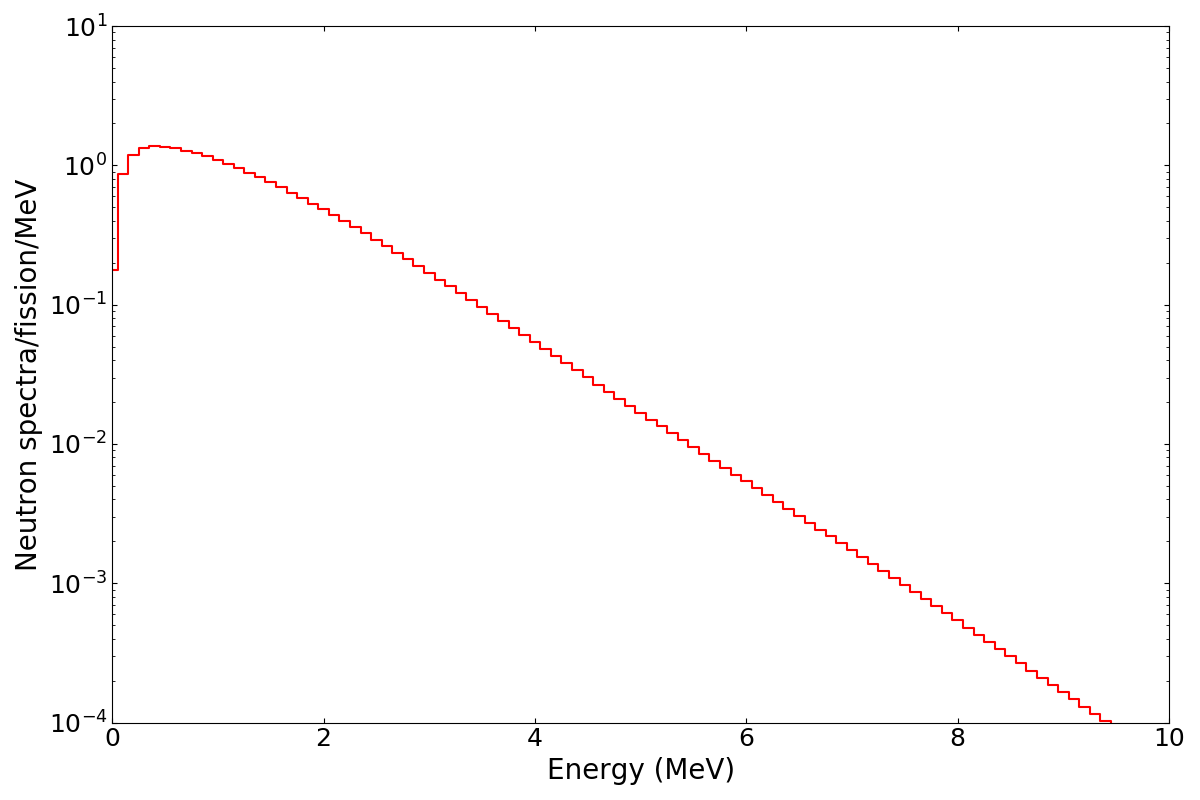Consistently calculated with the independent yield

Prompt neutron spectrum; prompt gamma spectrum

Particle multiplicities: P($\nu$), P($N_\gamma$)

## Uncertainties & Correlations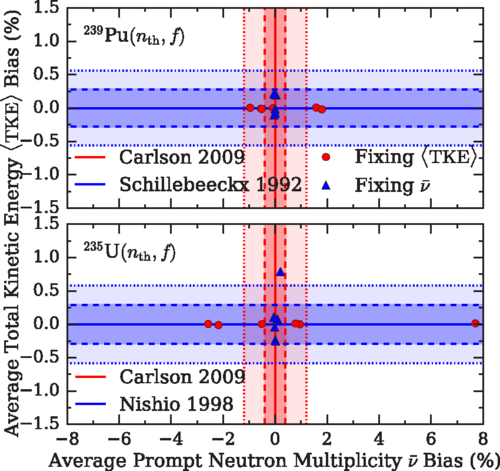Statistical & systematic uncertainties from experiment

Propagation of parameter uncertainties

Model defects

Jaffke et al. PRC 97 (2018)

## Special thanks to

The Nuclear Data Team @ LANL

P. Jaffke, T. Kawano, A. Lovell, I. Stetcu, P. Talou, M. Verriere
& many more...

Postdoc T-2 Staff XCP-5 Staff

## Summary

Current fission evaluations have not been updated in years

and are further lacking for modern applications

An effort to produce an improved fission yield evaluation is underway

• provide consistency between yields, n's & $\gamma$'s, and decay data